Next: Correcting for Staggered Leapfrog Up: Scale Factor Evolution Previous: Scale Factor Evolution

## The Scale Factor Equation

The equation for the scale factor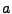is derived from the Friedmann equations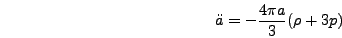(6.19)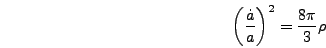(6.20)

For a set of scalar fields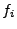in an FRW universe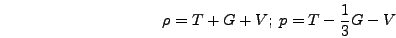(6.21)

where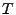,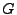, andare kinetic (time derivative), gradient, and potential energy respectively with(6.22)

Equations (6.19) and (6.20) and the field evolution equations form an overdetermined system. In principle either scale factor equation could be used but in practice it is easiest to combine them so as to eliminate the time derivative termbecause in the staggered leapfrog algorithm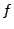andare known at different times. Eliminatingwe get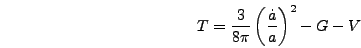(6.23)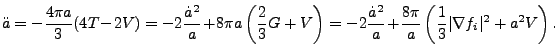(6.24)

To convert to program variables note that(6.25)

so the scale factor equation becomes(6.26)Next: Correcting for Staggered Leapfrog Up: Scale Factor Evolution Previous: Scale Factor Evolution